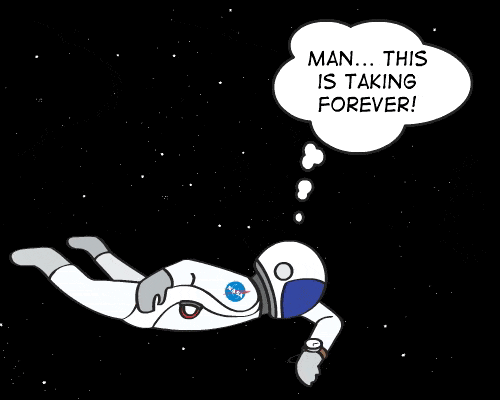# Miranda's Cliffs: Surviving a 12 Mile High Fall

• News
• zuz

#### zuz

If you fell off one of Miranda's 12 mile high cliffs. what would happen? Would you die? Assuming you are wearing an adequate spacesuit.The jumper would max out at around only 90 miles per hour and might even be able to land safely with some sort of futuristic airbag!

The fall would take so much more time because Miranda is much smaller than Earth. That means it has less gravity. In fact, the gravity is only 0.008 times as strong there as compared to Earth.
-NASA

•Wrichik Basu, russ_watters and jim mcnamara
0.079 m/s² is the value given here:
https://en.wikipedia.org/wiki/Miranda_(moon)
as the acceleration due to gravity on the surface of Miranda.

Do you know to figure out the velocity at impact? This, in turn, gives you an approximate value for impulse. Sort of the amount "wham" you experience from impact. This would be what you have to survive.

@Keith_McClary gave you a nice answer using cartoons. Maybe that answers it.

You should also realize humans have limits that vary from person to person for surviving falls. Age is a big factor. Mass of the falling person. Bone density. Fitness. Couch potatoes likely will fare worse.

Surface gravity is ##a=GM/R^2## and escape velocity is ##v=\sqrt{2GM/R}##. For Miranda, ##v=183m/s## and equatorial radius is 235,800m. [That's the data I found at https://solarviews.com/eng/miranda.htm ] so we can calculate the surface gravity from the radius and escape velocity as ##a= v^2/2R = 33,489/(2\cdot 235800) = 0.07101145 m/s^2##. The surface gravity of Earth being ##9.80665 m/s^2## gives us a ratio of 0.00724.

So, since equivalent speeds (of impact) correspond to equivalent kinetic energies and that would correspond to equivalent potential energies of falling (mass times acceleration times distance fallen) we see that the 12 mile fall or 19,312 meter fall on Miranda equates to a 139.84 meter (459.8 foot) fall on Earth, (about 42 stories in standard building height). Not something I'd be willing to experience.

•Wrichik Basu and jim mcnamara